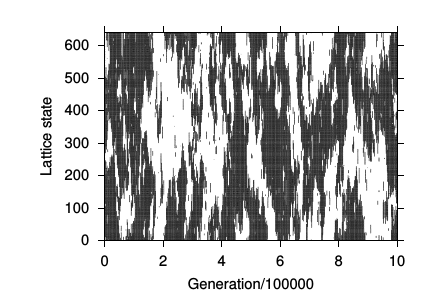# Neighborhood Approximations for Non-Linear Voter Models

## Authors: Frank Schweitzer and Laxmidhar Behera

Entropy (2015)

### Abstract

Non-linear voter models assume that the opinion of an agent depends on the opinions of its neighbors in a non-linear manner. This allows for voting rules different from majority voting. While the linear voter model is known to reach consensus, non-linear voter models can result in the coexistence of opposite opinions. Our aim is to derive approximations to correctly predict the time dependent dynamics, or at least the asymptotic outcome, of such local interactions. Emphasis is on a probabilistic approach to decompose the opinion distribution in a second-order neighborhood into lower-order probability distributions. This is compared with an analytic pair approximation for the expected value of the global fraction of opinions and a mean-field approximation. Our reference case is averaged stochastic simulations of a one-dimensional cellular automaton. We find that the probabilistic second-order approach captures the dynamics of the reference case very well for different non-linearities, i.e., for both majority and minority voting rules, which only partly holds for the first-order pair approximation and not at all for the mean-field approximation. We further discuss the interesting phenomenon of a correlated coexistence, characterized by the formation of large domains of opinions that dominate for some time, but slowly change.